# Single Line to Ground Fault

Consider a 3-phase system with an earth neutral system. Let a single line to ground fault occur on the red phase as shown in the fig below. It is clear from this figure that: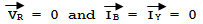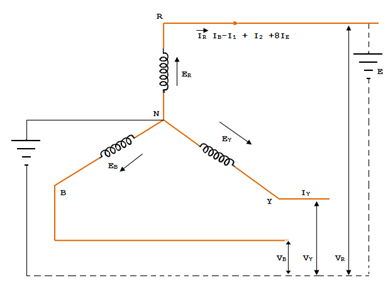The sequence current in the red phase in terms of line currents shall be: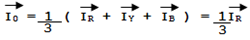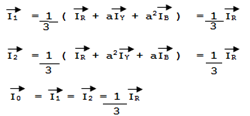# Single Line to Ground Fault Current

First of all, expression for fault current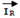will be derived. Let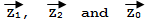be the positive, negative and zero sequence impedances of the generator respectively. Consider the closed loop NREN, As the sequence currents produce voltage drops due to their respective sequence impedances only, we have,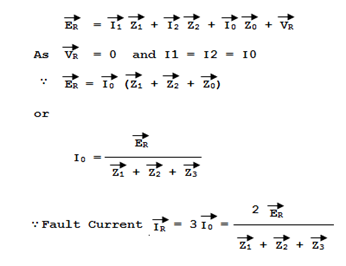## Explanation of experiment for Single Line to Ground Fault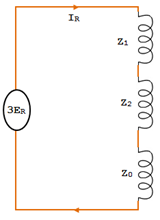The figure shows that the equivalent circuit for single line to ground fault from which fault current may be calculated is as given in the figure. It is clear that the fault current is obtained by connecting the phase sequence impedances in series across an imaginary generator of voltage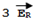. This is a wonderful part of the method of symmetrical and makes  the analysis easy and interesting . In fact this, method permits to bring any unsymmetrical fault into a simple circuit of interconnection of sequence impedances appropriate to the fault condition prevailing. The assumption made in arriving at experiment  is that the fault impedance is zero. However, if the fault impedance is Ze, then expression for the fault current becomes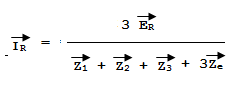It may be noted here that if the neutral is not grounded, then zero sequence impedance will be infinite and the fault current is zero. This is expected because now no path exists for the flow of fault current.

### Phase Voltages at fault

Now let us calcualte the phase voltages at fault (i.e., voltage between each line and fault). Since the generated e.m.f system is of positive sequence only, the sequence components of e.m.f. in R-phase are: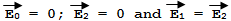The sequence voltages at the fault for R-phase are: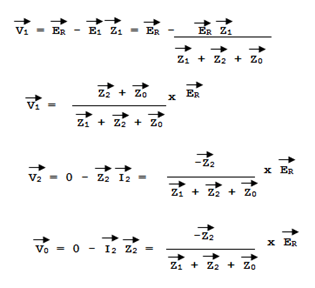It can be readily seen that this is expressed because R-Phase is shorted to ground. The phase voltages at fault are :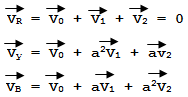#### Summary of results :

For Single line to ground fault  (R – Phase)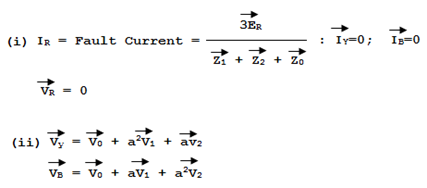2 CommentsComments

#### Read Comment Policy We have Zero Tolerance to Spam. Chessy Comments and Comments with Links will be deleted immediately upon our review.

1.The assumption made in arriving at experiment is that the fault impedance is zero. However, if the fault impedance is Ze, then expression for the fault current becomes. PEGACLSA74V1-A dumps

2.This particular papers fabulous, and My spouse and i enjoy each of the perform that you have placed into this. I’m sure that you will be making a really useful place. I has been additionally pleased. Good perform! Magnitude 9.o